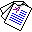# Coordination in Answer Set Programming

## Chiaki Sakama and Katsumi Inoue

ACM Transactions on Computational Logic, vol.9(2), Article No.9, 2008.

## Abstract

This paper studies a semantics of multiple logic programs, and synthesizes a program having such a collective semantics. More precisely, the following two problems are considered: given two logic programs P1 and P2, which have the collections of answer sets AS(P1) and AS(P2), respectively; (i) find a program Q which has the set of answer sets such that AS(Q)=AS(P1) ∪ AS(P2); (ii) find a program R which has the set of answer sets such that AS(R)=AS(P1) ∩ AS(P2). A program \$Q\$ satisfying the condition (i) is called generous coordination of P1 and P2; and R satisfying (ii) is called rigorous coordination of P1 and P2. Generous coordination retains all of the answer sets of each program, but permits the introduction of additional answer sets of the other program. By contrast, rigorous coordination forces each program to give up some answer sets, but the result remains within the original answer sets for each program. Coordination provides a program that reflects the meaning of two or more programs. We provide methods for constructing these two types of coordination and address its application to logic-based multi-agent systems.Full Paper (Pdf 223K) © ACM